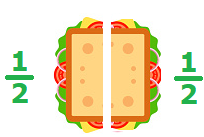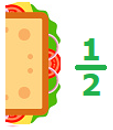# Math > Year 2

## Recognizing

A half is one of two equal parts.

Example
Here we have one whole sandwich.If we cut a sandwich into 2 equal parts, we’ll get two halves.Half of the sandwich is one of its two equal parts.To find of something, we split it into two equal parts.

For example, here are 8 gift boxes.To find half of it, we will divide them into two equal parts.Hence, to find one half, we divide by 2.
of 8 = 8 ÷ 2 = 4

Let's try a question.

If James eats half of the given sandwiches, how many sandwiches are left?Total number of sandwiches = 10

Fraction of the sandwiches eaten by James =

of 10

To find half of it, we will divide them into two equal parts.

Hence, to find one half, we divide by 2.

of 10

= 10 ÷ 2

= 5

//